# How To Optimize Images With HTML5 Canvas

Sergey Chikuyonok is a Russian front-end web-developer and writer with a big passion on optimization: from images and JavaScript effects to working process and … More about Sergey ↬

Weekly tips on front-end & UX.
Trusted by 176.000 folks.

Quick summary ↬ Images have always been the heaviest component of websites. Even if high-speed Internet access gets cheaper and more widely available, websites will get heavier more quickly. If you really care about your visitors, then spend some time deciding between good-quality images that are bigger in size and poorer-quality images that download more quickly. And keep in mind that modern Web browsers have enough power to enhance images right on the user’s computer. In this article, I’ll demonstrate one possible solution.

Let’s refer to an image that I came across recently in my job. As you can see, this image is of stage curtains and has some (intentional) light noise:

Optimizing an image like this would be a real pain because it contains a lot of red (which causes more artifacts in JPEG) and noise (which creates awful artifacts in JPEG and is bad for PNG packing). The best optimization I could get for this image was 330 KB JPEG, which is quite much for a single image. So, I decided to do some experiments with image enhancement right in the user’s browser.

More after jump! Continue reading below ↓

If you look closely at this image, you’ll see that it consists of two layers: the noise and the stage curtains. If we remove the noise, then the image shrinks to 70 KB in JPEG, which is really nice. Thus, our goal becomes to pass a noiseless image to the user and then add noise to the image right in the Web browser. This will greatly reduce the downloading time and make the Web page perform better.

In Photoshop, generating monochromatic noise is very easy: just go to `Filter``Noise``Add Noise`. But in the original image, the noise actually darkens some pixels (i.e. there are no white pixels). This brings a new challenge: to apply a noise layer with the “Multiply” blending mode on the stage image.

## HTML5 Canvas

All modern Web browsers support the canvas element. While early canvas implementations offered only a drawing API, modern implementations allow authors to analyze and manipulate every image pixel. This can be done with the ImageData interface, which represents an image data’s width, height and array of pixels.

The canvas pixel array is a plain array containing each pixels’s RGBa data. Here is what that data array looks like:

``````pixelData = [pixel1_red, pixel1_green,
pixel1_blue, pixel1_alpha, pixel2_red,
pixel2_green, pixel2_blue, pixel2_alpha, …];``````

Thus, an image data array contains `total_pixels×4` elements. For example, a 200×100 image would contain 200×100×4 = 80,000 elements in this array.

To analyze and manipulate individual canvas pixels, we have to get image data from it, then modify the pixel array and then put data back into the canvas:

``````// Coordinates of image pixel that we will modify
var x = 10, y = 20;

// Create a new canvas
var canvas = document.createElement('canvas');
canvas.width = canvas.height = 100;
document.body.appendChild(canvas);

// Get drawing context
var ctx = canvas.getContext('2d');

// Get image data
var imageData = ctx.getImageData(0, 0, canvas.width, canvas.height);

// Calculate offset for pixel
var offset = (x - 1 + (y - 1) * canvas.width) * 4;

// Set pixel color to opaque orange
imageData.data[offset]     = 255; // red channel
imageData.data[offset + 1] = 127; // green channel
imageData.data[offset + 2] = 0;   // blue channel
imageData.data[offset + 3] = 255; // alpha channel

// Put image data back into canvas
ctx.putImageData(imageData, 0, 0);``````

## Generating Noise

Once we know how to manipulate individual canvas pixels, we can easily create noise layer. A simple function that generates monochromatic noise might look like this:

``````function addNoise(canvas) {
var ctx = canvas.getContext('2d');

// Get canvas pixels
var imageData = ctx.getImageData(0, 0, canvas.width, canvas.height);
var pixels = imageData.data;

for (var i = 0, il = pixels.length; i < il; i += 4) {
var color = Math.round(Math.random() * 255);

// Because the noise is monochromatic, we should put the same value in the R, G and B channels
pixels[i] = pixels[i + 1] = pixels[i + 2] = color;

// Make sure pixels are opaque
pixels[i + 3] = 255;
}

// Put pixels back into canvas
ctx.putImageData(imageData, 0, 0);
}

// Set up canvas
var canvas = document.createElement('canvas');
canvas.width = canvas.height = 200;
document.body.appendChild(canvas);

The result could look like this: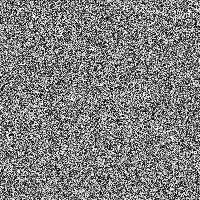Pretty good for starters. But we can’t just create a noise layer and place it above the scene image. Rather, we should blend it in “Multiply” mode.

## Blend Modes

Anyone who has worked with Adobe Photoshop or any other advanced image editor knows what layer blend modes are: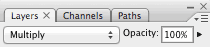Some folks regard image blend modes as some sort of rocket science, but in most cases the algorithms behind them are pretty simple. For example, here’s what the Multiply blending algorithm looks like:

``(colorA * colorB) / 255``

That is, we have to multiply two colors (each channel’s value) and divide it by 255.

Let’s modify out code snippet: load the image, generate the noise and apply it using the “Multiply” blend mode:

``````// Load image. Waiting for onload event is important
var img = new Image;
};
img.src = "stage-bg.jpg";

var canvas = document.createElement('canvas');
canvas.width = img.width;
canvas.height = img.height;

var ctx = canvas.getContext('2d');

// Draw image on canvas to get its pixel data
ctx.drawImage(img, 0, 0);

// Get image pixels
var imageData = ctx.getImageData(0, 0, canvas.width, canvas.height);
var pixels = imageData.data;

for (var i = 0, il = pixels.length; i < il; i += 4) {
// generate "noise" pixel
var color = Math.random() * 255;

// Apply noise pixel with Multiply blending mode for each color channel
pixels[i] =     pixels[i] * color / 255;
pixels[i + 1] = pixels[i + 1] * color / 255;
pixels[i + 2] = pixels[i + 2] * color / 255;
}

ctx.putImageData(imageData, 0, 0);
document.body.appendChild(canvas);
}``````

The result will look like this: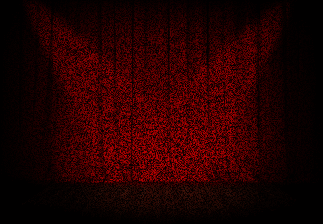Looks nice, but the noise is very rough. We have to apply transparency to it.

## Alpha Compositing

The process of combining two colors with transparency is called “Alpha compositing.” In the simplest case of compositing, the algorithm would look like this:

``colorA * alpha + colorB * (1 - alpha)``

Here, `alpha` is the composition coefficient (transparency) from 0 to 1. Choosing which color will be the background (`colorB`) and which will be the overlay (`colorA`) is important. In this case, the background will be the curtains image, and the noise will be the overlay.

Let’s add one more argument for the `addNoise()` function, which will control the alpha blending and modify the main function to respect transparency while blending images:

``````var img = new Image;
addNoise(img, 0.2); // pass 'alpha' argument
};
img.src = "stage-bg.jpg";

function addNoise(img, alpha) { // new 'alpha' argument
var canvas = document.createElement('canvas');
canvas.width = img.width;
canvas.height = img.height;

var ctx = canvas.getContext('2d');
ctx.drawImage(img, 0, 0);

var imageData = ctx.getImageData(0, 0, canvas.width, canvas.height);
var pixels = imageData.data, r, g, b;

for (var i = 0, il = pixels.length; i < il; i += 4) {
// generate "noise" pixel
var color = Math.random() * 255;

// Calculate the target color in Multiply blending mode without alpha composition
r = pixels[i] * color / 255;
g = pixels[i + 1] * color / 255;
b = pixels[i + 2] * color / 255;

// alpha compositing
pixels[i] =     r * alpha + pixels[i] * (1 - alpha);
pixels[i + 1] = g * alpha + pixels[i + 1] * (1 - alpha);
pixels[i + 2] = b * alpha + pixels[i + 2] * (1 - alpha);
}

ctx.putImageData(imageData, 0, 0);
document.body.appendChild(canvas);
}``````

The result is exactly what we want: a noise layer applied to the background image in Multiply blending mode, with a transparency of 20%: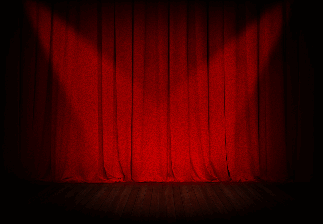## Optimization

While the resulting image looks perfect, the performance of this script is pretty poor. On my computer, it takes about 300 milliseconds (ms). On the average user’s computer, it could take even longer. So, we have to optimize this script. Half of the code uses browser API calls such as for creating the canvas, getting the image data and sending it back, so we can’t do much with that. The other half is the main loop for applying noise to the stage image, and it can be perfectly optimized.

Our test image size is 1293×897, which leads to 1,159,821 loop iterations. A pretty big number, so even small modifications could lead to significant performance boosts.

For example, in this cycle we calculated `1 - alpha` three times, but this is a static value. We should define a new variable outside of the `for` loop:

``var alpha1 = 1 - alpha;``

And then we would replace all occurrences of `1 - alpha` with `alpha1`.

Next, for the noise pixel generation, we use the `Math.random() * 255` formula. But a few lines later, we divide this value by 255, so `r = pixels[i] * color / 255`. Thus, we have no need to multiply and divide; we just use a random value. Here’s what the main loop looks like after these tweaks:

``````var alpha1 = 1 - alpha;
for (var i = 0, il = pixels.length; i < il; i += 4) {
// generate "noise" pixel
var color = Math.random();

// Calculate the target color in Multiply blending mode without alpha composition
r = pixels[i] * color;
g = pixels[i + 1] * color;
b = pixels[i + 2] * color;

// Alpha compositing
pixels[i] =     r * alpha + pixels[i] * alpha1;
pixels[i + 1] = g * alpha + pixels[i + 1] * alpha1;
pixels[i + 2] = b * alpha + pixels[i + 2] * alpha1;
}``````

After these little optimizations, the `addNoise()` function runs at about 240 ms (a 20% boost).

Remember that we have more than a million iterations, so every little bit counts. In the main loop, we’re accessing the `pixels` array twice: once for blending and once for alpha compositing. But array access is too resource-intensive, so we need to use an intermediate variable to store the original pixel value (i.e. we access the array once per iteration), like so:

``````var alpha1 = 1 - alpha;
var origR, origG, origB;

for (var i = 0, il = pixels.length; i < il; i += 4) {
// generate "noise" pixel
var color = Math.random();

origR = pixels[i]
origG = pixels[i + 1];
origB = pixels[i + 2];

// Calculate the target color in Multiply blending mode without alpha composition
r = origR * color;
g = origG * color;
b = origG * color;

// Alpha compositing
pixels[i] =     r * alpha + origR * alpha1;
pixels[i + 1] = g * alpha + origG * alpha1;
pixels[i + 2] = b * alpha + origB * alpha1;
}``````

This reduces the execution of this function down to 200 ms.

## Extreme Optimization

An attentive user would notice that the stage curtains are red. In other words, the image data is defined in the red channel only. The green and blue ones are empty, so there’s no need for them in the calculations:

``````for (var i = 0, il = pixels.length; i < il; i += 4) {
// generate "noise" pixel
var color = Math.random();

origR = pixels[i]

// Calculate the target color in Multiply blending mode without alpha composition
r = origR * color;

// Alpha compositing
pixels[i] = r * alpha + origR * alpha1;
}``````

With some high-school algebra, I came up with this formula:

``````for (var i = 0, il = pixels.length; i < il; i += 4) {
pixels[i] = pixels[i] * (Math.random() * alpha + alpha1);
}``````

And the overall execution of the function is reduced to 100 ms, one-third of the original 300 ms, which is pretty awesome.

The `for` loop contains just one simple calculation, and you might think that we can do nothing more. Actually, we can.

During the execution of the loop, we calculate the random pixel value and apply it to original one. But we don’t need to compute this random pixel on each iteration (remember, we have more than a million of them!). Rather, we can pre-calculate a limited set of random values and then apply them to original pixels. This will work because the generated value is… well, random. There’s no repeating patterns of special cases — just random data.

The trick is to pick the right value’s array size. It should be large enough to not produce visible repeating patterns on the image and small enough to be generated at a reasonable speed. During my experiments, the best random value’s array length was 3.73 of the image width.

Now, let’s generate an array with random pixels and then apply them to original image:

``````// Pick the best array length
var rl = Math.round(ctx.canvas.width * 3.73);
var randoms = new Array(rl);

// Pre-calculate random pixels
for (var i = 0; i < rl; i++) {
randoms[i] = Math.random() * alpha + alpha1;
}

// Apply random pixels
for (var i = 0, il = pixels.length; i < il; i += 4) {
pixels[i] = pixels[i] * randoms[i % rl];
}``````

This will cut down the execution time to 80 ms in Webkit browsers and have a significant boost in Opera. Also, Opera slows down in performance when the image data array contains float values, so we have to round them with fast bit-wise `OR` operator.

The final code snippet looks like this:

``````var img = new Image;
addNoise(img, 0.2); // pass 'alpha' argument
};
img.src = "stage-bg.jpg";

var canvas = document.createElement('canvas');
canvas.width = img.width;
canvas.height = img.height;

var ctx = canvas.getContext('2d');
ctx.drawImage(img, 0, 0);

var imageData = ctx.getImageData(0, 0, canvas.width, canvas.height);
var pixels = imageData.data;
var alpha1 = 1 - alpha;

// Pick the best array length
var rl = Math.round(ctx.canvas.width * 3.73);
var randoms = new Array(rl);

// Pre-calculate random pixels
for (var i = 0; i < rl; i++) {
randoms[i] = Math.random() * alpha + alpha1;
}

// Apply random pixels
for (var i = 0, il = pixels.length; i < il; i += 4) {
pixels[i] =  (pixels[i] * randoms[i % rl]) | 0;
}

ctx.putImageData(imageData, 0, 0);
document.body.appendChild(canvas);
}``````

This code executes in about 80 ms on my laptop in Safari 5.1 for an image that is 1293×897, which is really fast. You can see the result online.

## The Result

In my opinion, the result is pretty good:

• The image size was reduced from 330 KB to 70 KB, plus 1 KB of minified JavaScript. Actually, the image could be saved at a lower quality because the noise might hide some JPEG artifacts.
• This is a perfectly valid progressive enhancement optimization. Users with modern browsers will see a highly detailed image, while users with older browsers (such as IE 6) or with JavaScript disabled will still be able to see the image but with less detail.

The only downside of this approach is that the final image should be calculated each time the user visits the Web page. In some cases, you can store the final image as `data:url` in `localStorage` and restore it the next time the page loads. In my case, the size of the final image is 1.24 MB, so I decided not to store it and instead spend the default 5 MB of local storage on more useful data.

### Blend Modes Playground

I’ve created a small playground that you can use as a starting point for your experiments and optimizations. It contains most Photoshop blend modes and opacity control. Feel free to copy any code found on this playground into your pages.

## Conclusion

The technique you’ve just learned can be used in many different ways on modern Web pages. You don’t need to create many heavy, detailed images and force users to download them. Highlights, shades, textures and more can all be generated very quickly right in the user’s browser.

But use this technique wisely. Putting many unoptimized image generators on a single page could cause the browser to hang for a few seconds. You shouldn’t use this technique if you don’t really understand how it works or know what you’re doing. Always prioritize performance ahead of cool technology.

Also, note that the curtains image in this article is for demonstration purposes only. You can achieve almost the same result — a noisy image — just by placing a semi-transparent noise texture above the scene image, as shown in this example. Anyway, the point of this article was to show you how to enhance images right in the Web browser while keeping their size as small as possible.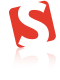(al)